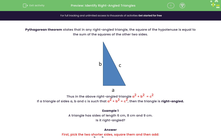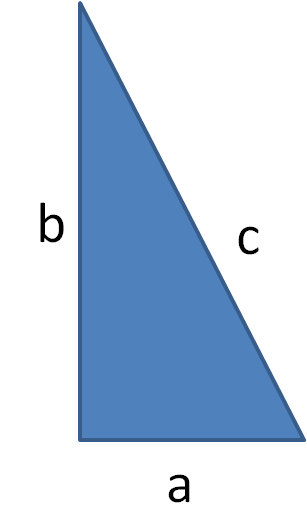# Identify Right-Angled Triangles

In this worksheet, students will use the converse of Pythagoras' Theorem to determine whether or not a triangle is right-angled.Key stage:  KS 3

Curriculum topic:   Geometry and Measures

Curriculum subtopic:   Solve Right-Angled Triangle Problems

Popular topics:   Geometry worksheets

Difficulty level:#### Worksheet Overview

Pythagoras' theorem states that in any right-angled triangle, the square of the hypotenuse is equal to the sum of the squares of the other two sides.Thus in the above right-angled triangle a2 + b2  = c2

If a triangle of sides a, b and c is such that a2 + b2 = c2, then the triangle is right-angled.

Example 1

A triangle has sides of length 6 cm, 8 cm and 9 cm.

Is it right-angled?

First, pick the two shorter sides, square them and then add:

62 + 82 = 36 + 64 = 100

Then square the longest side:

92 = 81

So 62 + 82 ≠ 92 and the triangle is therefore not right-angled.

Example 2

A triangle has sides of length 5 cm, 13 cm and 12 cm.

Is it right-angled?

First, pick the two shorter sides, square them and then add:

52 + 122 = 25 + 144 = 169

Then square the longest side:

132 = 169

So 52 + 122 = 132 and the triangle is therefore right-angled.

Don't worry too much if you feel unsure or overloaded with information.

We will work through ten questions together so that you feel super confident

### What is EdPlace?

We're your National Curriculum aligned online education content provider helping each child succeed in English, maths and science from year 1 to GCSE. With an EdPlace account you’ll be able to track and measure progress, helping each child achieve their best. We build confidence and attainment by personalising each child’s learning at a level that suits them.

Get started